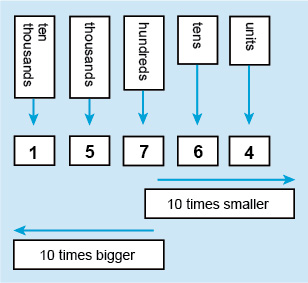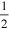Succeed with maths – Part 1

Start this free course now. Just create an account and sign in. Enrol and complete the course for a free statement of participation or digital badge if available.

Free course

# 4 Understanding numbers

To start your exploration of numbers you are going to concentrate on whole numbers or what you might also think about as the numbers that you count with.

Every number that we see around us is made up of a series of digits, such as 1302 (one thousand, three hundred and two). The value that each digit has depends not only on what that digit is but also on its place, or position, in a number. This allows you to represent any number that you want by using only 10 digits (0, 1, 2, 3 etc.).

Each place in a number has a value of ten times the place to its right, starting with ones or more properly called units, then tens, hundreds, thousands and so on. So in 1302, the 2 is in the units place, the 0 is in the tens place, the 3 is in the hundreds place and the 1 is in the thousands place. This means that the 3 has a value of 300 and the 1 has a value of 1000.

If you now rearrange the digits in your first number they will have different values and you’ll have a new number. So, 3120 has all the same digits but now the 3 is 3000, rather than 300 as it has moved one place to the left.

Have a look at this next example:Figure 10 Place value

You can see in the illustration above that the following is true:

• 1 is in the ten thousands place
• 5 is in the thousands place
• 7 is in the hundreds place
• 6 is in the tens place
• 4 is in the units place.

… and the number is 15 764, which is spoken as ‘fifteen thousand, seven hundred and sixty-four’.

Using this system you can write and understand any whole number but as you are probably aware numbers are not limited to only whole numbers. You also need ways to represent parts of whole numbers. The two ways that you do this is by using either decimal numbers, like 3.25, or fractions, such as. You’ll start with a look at decimal numbers, before taking a very brief look at fractions and how these to relate to decimal numbers. The real work on fractions will be left for Weeks 3 and 4, so this is just a gentle introduction!